##### Quantum Physics For Dummies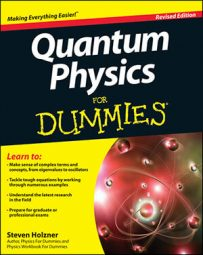In quantum physics, when you look at the spin eigenstates and operators for particles of spin 1/2 in terms of matrices, there are only two possible states, spin up and spin down.

The eigenvalues of the S2 operator are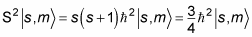and the eigenvalues of the Sz operator are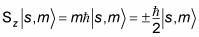You can represent these two equations graphically as shown in the following figure, where the two spin states have different projections along the z axis.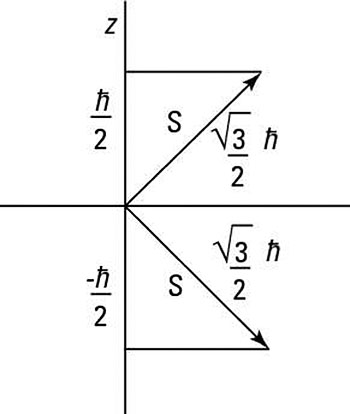Spin magnitude and z projection.

In the case of spin 1/2 matrices, you first represent the eigenstate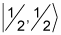like this: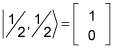And the eigenstate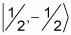looks like this: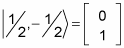Now what about spin operators like S2? The S2 operator looks like this in matrix terms: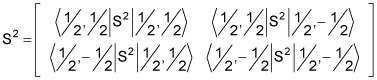And this works out to be the following: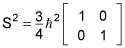Similarly, you can represent the Sz operator this way: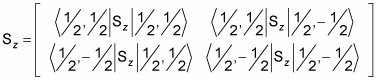This works out to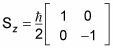Using the matrix version of Sz, for example, you can find the z component of the spin of, say, the eigenstate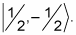Finding the z component looks like this: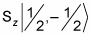Putting this in matrix terms gives you this matrix product: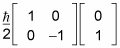Here’s what you get by performing the matrix multiplication: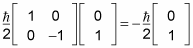And putting this back into ket notation, you get the following: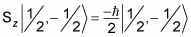How about the raising and lowering operators S+ and S? The S+ operator looks like this: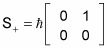And the lowering operator looks like this: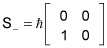Here it is in matrix terms: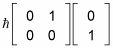Performing the multiplication gives you this: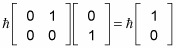Or in ket form, it’s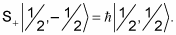Cool.